# 世界杯：用Python分析热门夺冠球队-(附源代码)

2018年，火热的世界杯即将拉开序幕。在比赛开始之前，我们不妨用 Python 来对参赛队伍的实力情况进行分析，并大胆的预测下本届世界杯的夺冠热门球队。

• 找出哪些队伍是首次进入世界杯的黑马队伍
• 找出2018年32强中之前已经进入过世界杯，但在世界杯上没有赢得过一场比赛的队伍

• window 7 系统
• python 3.6
• Jupyter Notebook
• pandas version 0.22.0

import pandas as pd
import matplotlib.pyplot as plt

%matplotlib inline
plt.style.use('ggplot')



• 日期
• 主队名称
• 客队名称
• 主队进球数 （不含点球）
• 客队进球数 （不含点球）
• 比赛的类型
• 比赛所在城市
• 比赛所在国家
• 是否中立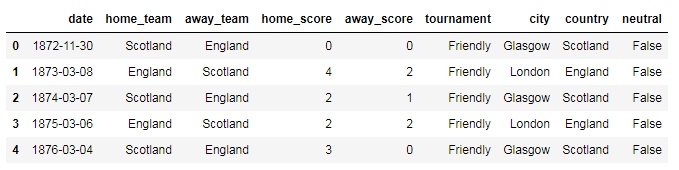## 1、 获取所有世界杯比赛的数据（不含预选赛）

df_FIFA_all = df[df['tournament'].str.contains('FIFA', regex=True)]
df_FIFA = df_FIFA_all[df_FIFA_all['tournament']=='FIFA World Cup']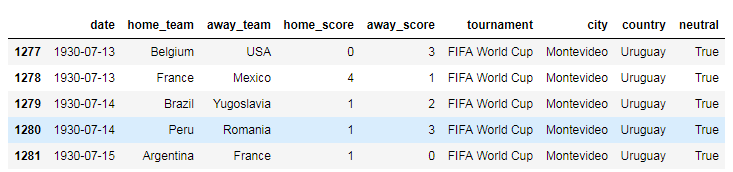df_FIFA.loc[:,'date'] = pd.to_datetime(df_FIFA.loc[:,'date'])
df_FIFA['year'] = df_FIFA['date'].dt.year
df_FIFA['diff_score'] = df_FIFA['home_score']-df_FIFA['away_score']
df_FIFA['win_team'] = ''
df_FIFA['diff_score'] = pd.to_numeric(df_FIFA['diff_score'])


# The first method to get the winners

df_FIFA.loc[df_FIFA['diff_score']> 0, 'win_team'] = df_FIFA.loc[df_FIFA['diff_score']> 0, 'home_team']
df_FIFA.loc[df_FIFA['diff_score']< 0, 'win_team'] = df_FIFA.loc[df_FIFA['diff_score']< 0, 'away_team']
df_FIFA.loc[df_FIFA['diff_score']== 0, 'win_team'] = 'Draw'

# The second method to get the winners

def find_win_team(df):
winners = []
for i, row in df.iterrows():
if row['home_score'] > row['away_score']:
winners.append(row['home_team'])
elif row['home_score'] < row['away_score']:
winners.append(row['away_team'])
else:
winners.append('Draw')
return winners

df_FIFA['winner'] = find_win_team(df_FIFA)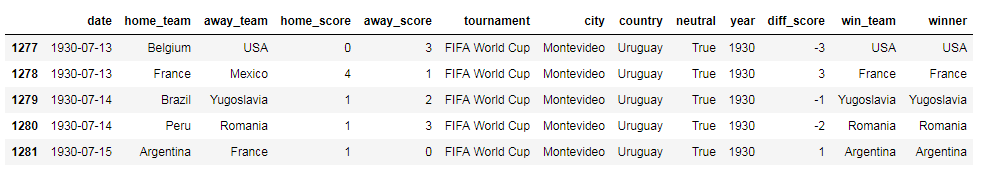## 2、 获取世界杯所有比赛的前20强数据情况

### 2.1 获取世界杯所有比赛获胜场数最多的前20强数据

s = df_FIFA.groupby('win_team')['win_team'].count()
s.sort_values(ascending=False, inplace=True)
s.drop(labels=['Draw'], inplace=True)


s.head(20).plot(kind='bar', figsize=(10,6), title='Top 20 Winners of World Cup')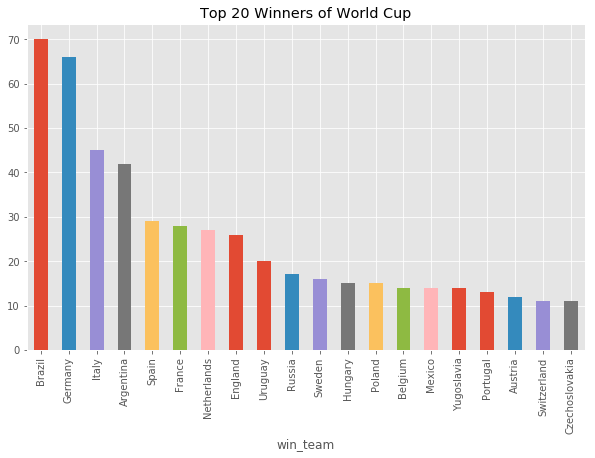s.sort_values(ascending=True,inplace=True)
s.tail(20).plot(kind='barh', figsize=(10,6), title='Top 20 Winners of World Cup')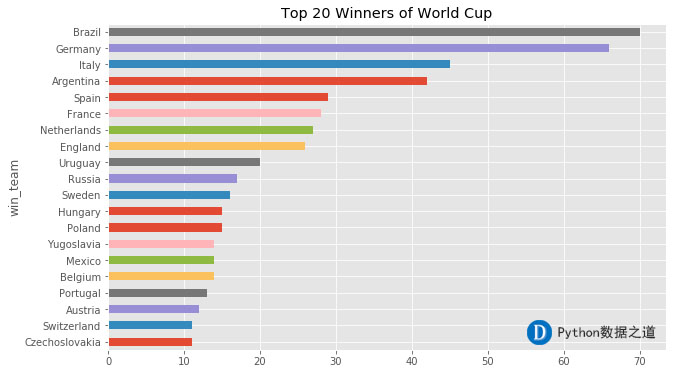s_percentage = s/s.sum()
s_percentage
s_percentage.tail(20).plot(kind='pie', figsize=(10,10), autopct='%.1f%%',
startangle=173, title='Top 20 Winners of World Cup', label='')s.get('China', default = 'NA')
s.get('Japan', default = 'NA')
s.get('Korea DPR', default = 'NA')
s.get('Korea Republic', default = 'NA')
s.get('Egypt', default = 'NA')


### 2.2 各个国家队进球总数量情况

df_score_home = df_FIFA[['home_team', 'home_score']]
column_update = ['team', 'score']
df_score_home.columns = column_update
df_score_away = df_FIFA[['away_team', 'away_score']]
df_score_away.columns = column_update
df_score = pd.concat([df_score_home,df_score_away], ignore_index=True)
s_score = df_score.groupby('team')['score'].sum()
s_score.sort_values(ascending=False, inplace=True)
s_score.sort_values(ascending=True, inplace=True)
s_score.tail(20).plot(kind='barh', figsize=(10,6), title='Top 20 in Total Scores of World Cup')## 3、2018年世界杯32强分析

2018年世界杯的分组情况如下：

team_list = ['Russia', 'Germany', 'Brazil', 'Portugal', 'Argentina', 'Belgium', 'Poland', 'France',
'Spain', 'Peru', 'Switzerland', 'England', 'Colombia', 'Mexico', 'Uruguay', 'Croatia',
'Denmark', 'Iceland', 'Costa Rica', 'Sweden', 'Tunisia', 'Egypt', 'Senegal', 'Iran',
'Serbia', 'Nigeria', 'Australia', 'Japan', 'Morocco', 'Panama', 'Korea Republic', 'Saudi Arabia']
for item in team_list:
if item not in s_score.index:
print(item)

out:
Iceland
Panama


df_top32 = df_FIFA[(df_FIFA['home_team'].isin(team_list))&(df_FIFA['away_team'].isin(team_list))]


### 3.1 自1872年以来，32强数据情况

s_32 = df_top32.groupby('win_team')['win_team'].count()
s_32.sort_values(ascending=False, inplace=True)
s_32.drop(labels=['Draw'], inplace=True)
s_32.sort_values(ascending=True,inplace=True)
s_32.plot(kind='barh', figsize=(8,12), title='Top 32 of World Cup since year 1872')df_score_home_32 = df_top32[['home_team', 'home_score']]
column_update = ['team', 'score']
df_score_home_32.columns = column_update
df_score_away_32 = df_top32[['away_team', 'away_score']]
df_score_away_32.columns = column_update
df_score_32 = pd.concat([df_score_home_32,df_score_away_32], ignore_index=True)
s_score_32 = df_score_32.groupby('team')['score'].sum()
s_score_32.sort_values(ascending=False, inplace=True)
s_score_32.sort_values(ascending=True, inplace=True)
s_score_32.plot(kind='barh', figsize=(8,12), title='Top 32 in Total Scores of World Cup since year 1872')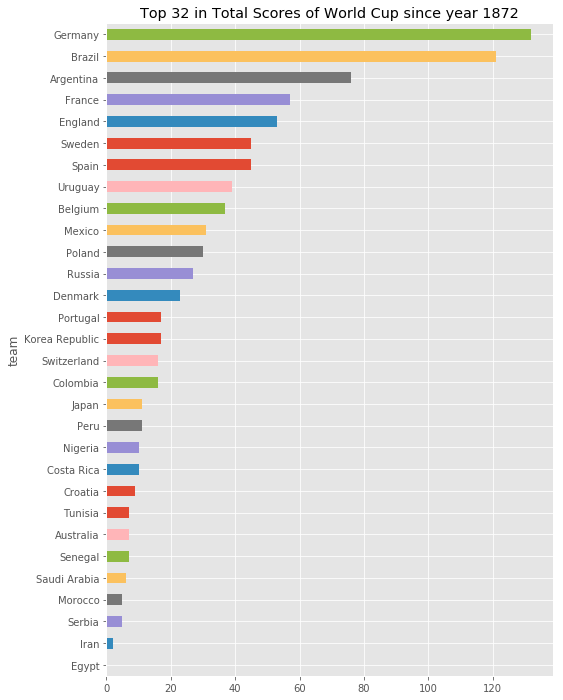### 3.2 自1978年以来，32强数据情况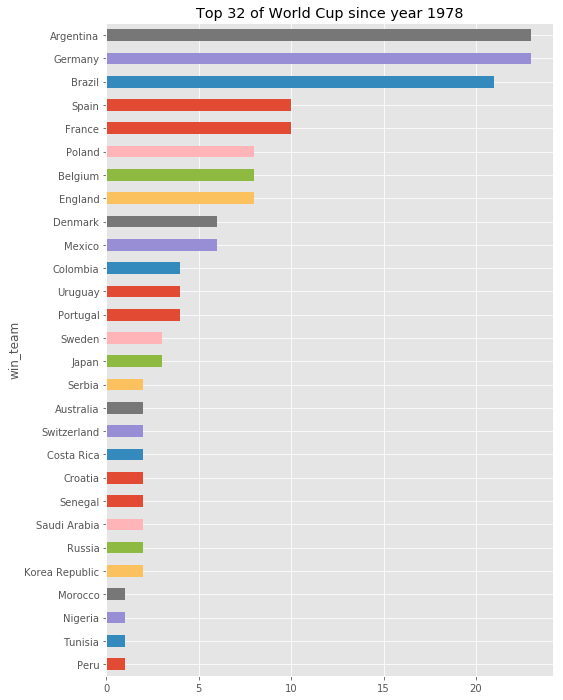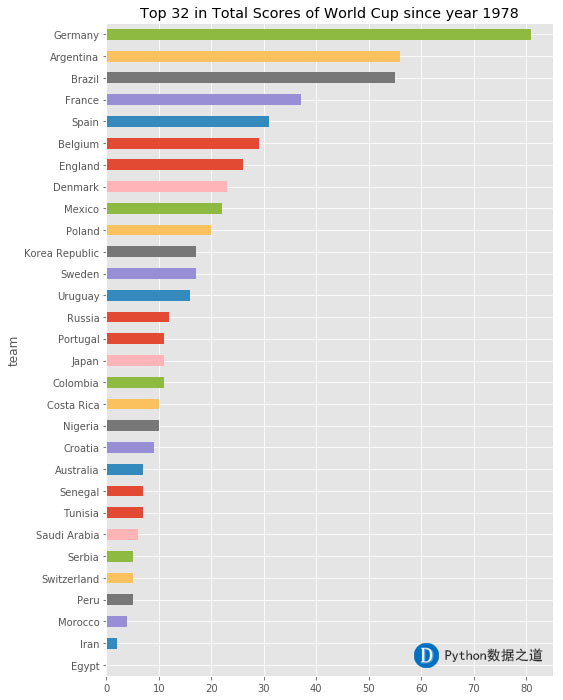### 3.3 自2002年以来，32强数据情况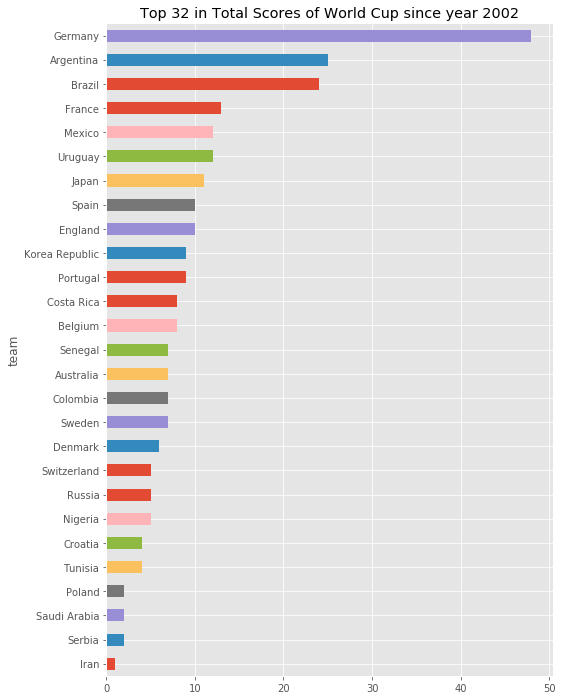## 4、综合结论

2018年世界杯的32支队伍，根据以往的世界杯比赛数据来看，预测前三强为 德国、阿根廷和巴西，其中德国队应该是夺冠的最大热门。

posted @ 2018-06-12 20:39  lemonbit  阅读(3099)  评论(5编辑  收藏  举报